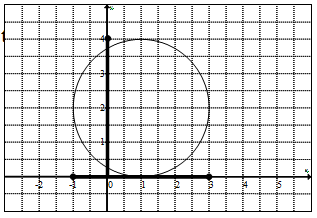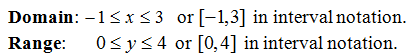## Pages

Showing posts with label domain. Show all posts
Showing posts with label domain. Show all posts

### Relations, Graphs, and Functions

In this section we will study functions in more depth and begin by defining a relation as any set of ordered pairs.
An example of a relation might look like { (2,3), (2,5), (0,1) }. Here we have a relation consisting of three ordered pairs or points on the Cartesian coordinate system. We are familiar with ordered pairs and usually see them denoted as (x, y).  Typically the x-value (the first component) will be the independent variable or input and the y-value (the second component) is the dependent variable or output.
The example { (2,3), (2,5), (0,1) } is NOT a function because the x-value 2 is assigned more than one y-value, namely 3 and 5. For every x-value there can be only one y-value. Next we define the domain as the set of x-values and the range as the set of y-values for which the relation is defined.
Example:
Tip: When looking at a list of ordered pairs, if there are repeating x-values then the relation is not a function.  This usually indicates that there is an input with multiple outputs. (This does not apply to the y-values)

Determine if the relations are functions.  If so state the domain and range.
Relations can consist of an infinite number of ordered pairs, in which case, making a big list would be impossible. A graph can represent a relation by considering it as a big list of ordered pairs.  Each dot on the graph represents an ordered pair (x, y).
Notice that if we can draw a vertical line that intersects the graph twice we will be able to identify one x-value with two corresponding y-values.  Therefore, it can not be a function.
Alternatively, if any vertical line crosses the graph only once then it does represent a function.

Use the vertical line test to determine whether or not the graph represents a function.
Be prepared to state the domain and range given the graph.  Remember to think of the graph as an infinite set of ordered pairs (x, y).  From the graph, determine the x-values and y-values. First shade in the domain and range then convert to interval notation.
It should be clear, at this point, that the circle shown is not a function.  Nonetheless, we still can determine the domain and range of the relation that it represents.YouTube Video:

---

### Simplifying Rational Expressions

Given a rational expression, the quotient of two polynomials, we will factor the numerator and denominator if we can and then cancel factors that are exactly the same.
When evaluating rational expressions, plug in the appropriate values either before simplifying or after, the result will be the same.  Although, it is more efficient to simplify first then evaluate.
We can see that when evaluating, the result will be the same whether or not we simplify first.  It turns out that not all numbers can be used when we evaluate.
The point is that not all real numbers will be defined in the above rational expression.  In fact, there are two restrictions to the domain, -2 and 3/5.  These values, when plugged in, will result in zero in the denominator.  Another way to say this is that the domain consists of all real numbers except for −2 and 3/5.

Tip: To find the restrictions, set each factor in the denominator equal to zero and solve. The factors in the numerator do not contribute to the list of restrictions.

Simplify and state the restrictions to the domain.

Even if the factor cancels it still contributes to the list of restrictions.  Basically, it is important to remember the domain of the original expression when simplifying. Also, we must use caution when simplifying, please do not try to take obviously incorrect shortcuts like this:

Since subtraction is not commutative, we must be alert to opposite binomial factors.  For example, 5 − 3 = 2 and 3 − 5 = −2. In general,
Simplify and state the restrictions to the domain.
At this point, we evaluate using function notation.
Video Examples on YouTube: